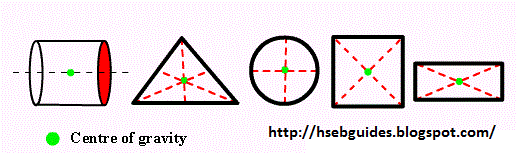# Torque- Centre Of Gravity

Definition
The tendency of a force to produce rotation in a body
about an axis is called torque or momentum of force.

Mathematical definition
Torque produced in a body is numerically equal to the product of force and moment arm.
Torque = (force ) ( moment arm)

Vectorial definition
Torque produced in a body is equal to the cross product of
force arm ( ) and the force ( ).
The turning effect of a force depends upon two factors:
Magnitude of force(F)
Magnitude of moment arm(r)
The torque about any axis is given by the product of force and moment arm.

Explanation
Consider a particle of mass ‘m’ which is acted upon a force. Let be the position vector of the particle which is also the position vector of the point of application of force. We can resolve this force into two rectangular components:

1. Parallel to the position vector i.e. Or Fcosq
2. Perpendicular to the position vector i.e. Or Fsinq

From figure, it is clear that can pull the particle but it cannot rotate it or it is unable to produce torque in the mass. It is the component which produces rotation in the mass.
Let r and be the magnitude of and respectively.
The magnitude of the torque produced about the centre 'O' is defined as:
Where q is the smaller angle between the positive direction of and
Using vector notation, above expression can also be written as:

Direction of torque
The direction of torque is directed along the normal to the plane formed by and as given by right-hand rule.

Sign convention
Positive torque:
If a body rotates about its axis in anti-clockwise direction, then the torque is taken positive.

Negative torque:
If the body rotates in the clockwise direction, then the torque is taken as negative.

Determinant from of torque
Let the position vector is = x i+ y j+ z k and the force= Fx i+ Fy j+ Fz k
The torque is given by:
Unit of torque
In SI system unit of torque is N-m ( Newton . Metre)
Dimension of torque
[L2MT-2]

Torque-center of gravity
Torque
The torque or moment of force can be defined as
The tendency of a force to produce rotation in a body
about an axis is called torque or moment of force.
The turning effect of a force depends upon two factors:
The magnitude of force(F)
Moment arm(r)
The torque about any axis is given by the product of force and moment arm

Positive torque:
If a body rotates about its axis in anti-clockwise direction, then the torque is taken positive.

Negative torque:
If the body rotates in the clockwise direction, then the torque is taken as negative.

Center of gravity
The center of a body is that point in the body through which the resultant forces due to the earth’s attraction posses and through which the whole weight of the body always acts.
Or
Center of gravity of a body is a point where total weight of the body is concentrated.
Every body posses a center of gravity and this is irrespective of the body. Its is not necessary that the center of gravity should be within the body, but it may also be situated in space outside the body. Example: center of gravity of a ring is at the center, which is in the space.
Center of gravity of different objects:

- Rectangle
Center of gravity of a rectangular is at the point of intersection of its diagonals

- Circle
Center of gravity of a circle is at its center.

- Square
Center of gravity of square is at the point of intersection of its diagonals.

- Regular bar
The center of gravity of a regular bar is at its geometrical center.

- Triangle
The center of gravity of a triangle is at the point of intersection of its medians.

- Cylinder
The center of gravity of a cylinder is at the axis of cylinder.